# Goniomentry and trigonometry - math word problems

1. Scalene triangleSolve the triangle: A = 50°, b = 13, c = 6
2. Two triangles SSA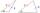Two triangles can be formed with the given information. Use the Law of Sines to solve the triangles. A = 59°, a = 13, b = 14
3. Find the areaFind the area of the triangle with the given measurements. Round the solution to the nearest hundredth if necessary. A = 50°, b = 30 ft, c = 14 ftAn airplane leaves an airport and flies to west 120 miles and then 150 miles in the direction S 44.1°W. How far is the plane from the airport (round to the nearest mile)?A ship travels 84 km on a bearing of 17°, and then travels on a bearing of 107° for 135 km. Find the distance of the end of the trip from the starting point, to the nearest kilometer.
6. Two forcesTwo forces with magnitudes of 25 and 30 pounds act on an object at angles of 10° and 100° respectively. Find the direction and magnitude of the resultant force. Round to two decimal places in all intermediate steps and in your final answer.
7. Supermarket cashiers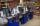When at the supermarket are opened only 2 cash people waiting in the front approximately 12 minutes. How many will shorten the average waiting time in a front where supermarket open another three cashiers?
8. Nine-gonCalculate the perimeter of a regular nonagon (9-gon) inscribed in a circle with a radius 13 cm.
9. Paper box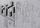Calculate the consumption of paper on the box-shaped quadrangular prism with rhombic footstall, base edge a=6 cm and the adjacent base edges forms an angle alpha = 60 °. Box height is 10 cm. How many m2 of the paper consumed 100 such boxes?
10. High wallI have a wall 2m high. I need a 15 degree angle (upward) to second wall 4 meters away. How high must the second wall?
11. FlowerbedFlowerbed has the shape of a truncated pyramid, the bottom edge of the base a = 10 m, the upper base b = 9 m. Deviation angle between edge and the base is alpha = 45°. What volume is needed to make this flowerbed? How many plants can be planted if 1 m2 =
12. Pyramid - angleCalculate the surface of regular quadrangular pyramid whose base edge measured 6 cm and the deviation from the plane of the side wall plane of the base is 50 degrees.How many square meters is needed to cover the tower the shape of regular quadrangular pyramid base edge 10 meters, if the deviation lateral edges from the base plane is 68 °? Calculate coverage waste 10%.
14. Circular sector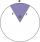I have a circular sector with a length 15 cm with an unknown central angle. It is inscribed by a circle with radius 5 cm. What is the central angle alpha in the circular sector?
15. Rhomboid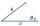The dimensions of the rhomboid sides are a= 5cm, b = 6 cm and the size of the angle at the vertex A is 60°. What is the length of side AC?
16. BevelI have bevel in the ratio 1:6. What is the angle and how do I calculate it?
17. Cross-country competition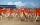Cross-country competition was attended by 76% of pupils of class 9.B. Class consis of 16 boys, two of whom did not run and 4 girls did not run. How many girls go to class 9.B?
18. Goat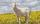Meadow is a circle with radius r = 19 m. How long must a rope to tie a goat to the pin on the perimeter of the meadow to allow goat eat half of meadow?
19. Sphere in coneA sphere of radius 3 cm desribe cone with minimum volume. Determine cone dimensions.
20. CuboidCuboid ABCDEFGH with 10 cm height has a base edge length 6 cm and 8 cm. Determine angle between body diagonal and the base plane (round to degrees).

Do you have an interesting mathematical word problem that you can't solve it? Submit math problem, and we can try to solve it.

We will send a solution to your e-mail address. Solved examples are also published here. Please enter the e-mail correctly and check whether you don't have a full mailbox.

Please do not submit problems from current active competitions such as Mathematical Olympiad, correspondence seminars etc...

Most natural application of trigonometry and trigonometric functions is a calculation of the triangles. Common and less common calculations of different types of triangles offers our triangle calculator. Word trigonometry comes from Greek and literally means triangle calculation.